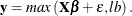### Example 13.3 Censored Data Models in PROC ENTROPY

Data available to an analyst might sometimes be censored, where only part of the actual series is observed. Consider the case in which only observations greater than some lower bound are recorded, as defined by the following process:Running ordinary least squares estimation on data generated by the preceding process is not optimal because the estimates are likely to be biased and inefficient. One alternative to estimating models with censored data is the tobit estimator. This model is supported in the QLIM procedure in SAS/ETS and in the LIFEREG procedure in SAS/STAT. PROC ENTROPY provides another alternative which can make it very easy to estimate such a model correctly.

The following DATA step generates censored data in which any negative values of the dependent variable, y, are set to a lower bound of 0.

data cens;
do t = 1 to 100;
x1 = 5 * ranuni(456);
x2 = 10 * ranuni(456);
y = 4.5*x1 + 2*x2 + 15 * rannor(456);
if( y<0 ) then y = 0;
output;
end;
run;


To illustrate the effect of the censored option in PROC ENTROPY, the model is initially estimated without accounting for censoring in the following statements:

title "Censored Data Estimation";
proc entropy data = cens gme primal;
priors intercept -32 32
x1        -15 15
x2        -15 15;
model y = x1 x2 /
esupports = (-25 1 25);
run;


Output 13.3.1: GME Estimates

 Censored Data Estimation

The ENTROPY Procedure

GME Variable Estimates
Variable Estimate Approx Std Err t Value Approx
Pr > |t|
x1 2.377609 0.000503 4725.98 <.0001
x2 2.353014 0.000255 9244.87 <.0001
intercept 5.478121 0.00188 2906.41 <.0001

The previous model is reestimated by using the CENSORED option in the following statements:

proc entropy data = cens gme primal;
priors intercept -32 32
x1        -15 15
x2        -15 15;
model y = x1 x2 /
esupports = (-25 1 25)
censored(lb = 0, esupports=(-15 1 15) );
run;


Output 13.3.2: Entropy Estimates

 Censored Data Estimation

The ENTROPY Procedure

GME Variable Estimates
Variable Estimate Approx Std Err t Value Approx
Pr > |t|
x1 4.429697 0.00690 641.85 <.0001
x2 1.46858 0.00349 420.61 <.0001
intercept 8.261412 0.0259 319.51 <.0001

The second set of entropy estimates are much closer to the true parameter estimates of 4.5 and 2. Since another alternative available for fitting a model of censored data is a tobit model, PROC QLIM is used in the following statements to fit a tobit model to the data:

proc qlim data=cens;
model y = x1 x2;
endogenous y ~ censored(lb=0);
run;


Output 13.3.3: QLIM Estimates

 Censored Data Estimation

The QLIM Procedure

Parameter Estimates
Parameter DF Estimate Standard Error t Value Approx
Pr > |t|
Intercept 1 2.979455 3.824252 0.78 0.4359
x1 1 4.882284 1.019913 4.79 <.0001
x2 1 1.374006 0.513000 2.68 0.0074
_Sigma 1 13.723213 1.032911 13.29 <.0001

For this data and code, PROC ENTROPY produces estimates that are closer to the true parameter values than those computed by PROC QLIM.